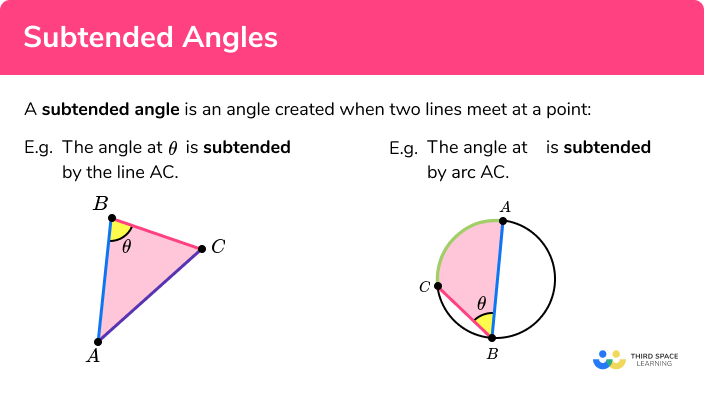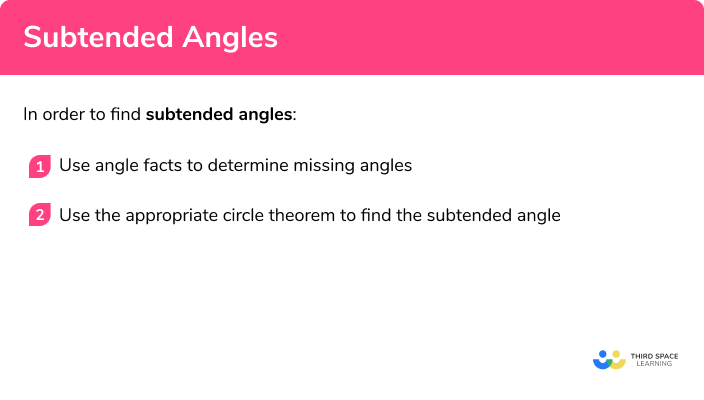# Subtended Angles

Here we will learn about subtended angles, including subtended angles of a line, curve and within polygons.

There are also subtended angles worksheets based on Edexcel, AQA and OCR exam questions, along with further guidance on where to go next if you’re still stuck.

## What is a subtended angle?

A subtended angle is the angle between two lines at a point.

For example,

The side AC subtends the angle θ from point B .

We can, therefore, describe a subtended angle as the angle made from a given point.

For circle theorems, a subtended angle is an angle within a circle that is created by two chords meeting at a point on the circumference of a circle.

The diagrams below show the angle subtended by arc AC from point B for two different circles.

### What is a subtended angle## How to find subtended angles

In order to find subtended angles:

1. Use angle facts to determine missing angles.
2. Use the appropriate circle theorem to find the subtended angle.

### How to find subtended anglesTangent of a circle is one of 7 circle theorems you will need to know. You may find it helpful to start with our main circle theorems page and then look in detail at the rest.

## Subtended angles examples

### Example 1: angles in the same segment

In the diagram, ∠BAC=70° , ∠ACB=40° . Find ∠ADC .

1. Use angle facts to determine missing angles.

As angles in a triangle total 180° , angle ABC = 180 - (70+40) = 70° .

2Use the appropriate circle theorem to find the subtended angle.

As angles in the same segment are equal and AC is a chord shared by the points B and D within the same segment, the angle ADC = angle ABC = 70° .

### Example 2: angles in the same segment

The diagram shows a circle with centre D and ∠CAD=42° . Find ∠ABC .

Triangle ACD is isosceles as AD and AC are radii. This means that angle ADC = 180 - (42 + 42) = 96° .

The angle at the centre is twice the angle at the circumference and so as the angles ABC and ADC are subtended by the same arc AC , angle ABC is half of angle ADC :

ABC = 96 ÷ 2 = 48° .

### Example 3: angles in a semicircle

The diagram shows a circle with centre C and ∠ABD=27° . Find ∠CAD .

Triangle ABC is isosceles as AC and BC are radii. Angle BAC = 27° .

The angle at the circumference of a semicircle is equal to 90 degrees as the line BD is the diameter. This means that angle CAD = 90 - 27 = 63° .

In the diagram, ∠CBD=43° and ∠CDB=22° . Find ∠BAD .

As angles in a triangle total 180° , angle BCD = 180 - (22+43) = 115° .

Opposite angles in a cyclic quadrilateral total 180° .

So, angle BAD = 180 - 115 = 65° .

### Example 5: alternate segment theorem

In the diagram, O is the centre, ∠ACB=58° and AD and BC are parallel. Find ∠CDF .

As lines AD and BC are parallel, we can state that angle CAD is alternate to angle BCA and so angle CAD = 58° .

The angle at A is subtended by the chord CD . The angle CDF is in the alternate segment to angle CAD so angle CDF = 58° .

### Example 6: angles in the same segment

In the diagram, ∠ABD=80° and ∠ADE=23° . Find ∠DCE .

For this problem, we need to use circle theorems to state missing angles.

Angle BAD = Angle BED = 90° as angles in a semicircle are 90° . This means that angle BDA = 180 - (90 + 80) = 10° .

This means that BDE = 33° and so DBE = 180 - (90+33) = 57° .

The angles at B and C are subtended by the chord DE . This means that they are in the same segment and so DCE = 57° .

### Common misconceptions

Make sure you know the other angle facts including:
• Angles on a straight line total 180°.
• Angles at a point total 360°.
• Angles in a triangle total 180°.
• Angles in a quadrilateral total 360°.
• Halving and doubling
By remembering the angle at the centre theorem incorrectly, the student could double the angle at the centre, or half the angle at the circumference.
Top tip: look at the angles. The angle at the centre is always larger than the angle at the circumference (this isn’t so obvious when the angle at the circumference is in the opposite segment).

• Angles are the same
Make sure that you know when two angles are equal. Look out for isosceles triangles and the angles in the same segment.
• A diameter or a chord?
The angle at the circumference is assumed to be 90° when the associated chord does not intersect the centre of the circle and so the diagram does not show a semicircle.

### Practice subtended angles questions

1. In the diagram, ∠BAD=83^{\circ} , ∠ABD=41^{\circ} . Find ∠ACB .83^{\circ}41^{\circ}56^{\circ}82^{\circ}ADB = 180 – (83+41) = 56^{\circ} (Angles in a triangle total 180^{\circ}

Angle ADC = Angle ACB (Angles in the same segment are equal)

ACB = 56^{\circ}

2. In the diagram, D is the centre, ∠ABC=38^{\circ} . Find ∠ACD .38^{\circ}52^{\circ}76^{\circ}104^{\circ}ADC = 38\times2 = 76^{\circ} (the angle at the centre is twice the angle at the circumference)

Triangle ACD is isosceles so angle ACD = (180 – 76)\div2 = 52^{\circ}

3. In the diagram, ∠ACB=53^{\circ} . Find ∠BAE .26.5^{\circ}37^{\circ}53^{\circ}90^{\circ}BE is a diameter

BAE = 90^{\circ}

4. In the diagram, ∠BCD=88^{\circ}, ∠ADE=49^{\circ}, ∠AED=78^{\circ} . Find ∠BAE .29^{\circ}53^{\circ}39^{\circ}44^{\circ}BAD = 180 – 88 = 92^{\circ} (opposite angles in a cyclic quadrilateral total 180^{\circ} )

DAE = 180 – (78+49) = 53^{\circ} (angles in a triangle total 180^{\circ} )

BAE = 92 – 53 = 29^{\circ}

5. In the diagram, with centre O , ∠ABE=58^{\circ} . Find ∠AOB .29^{\circ}32^{\circ}58^{\circ}116^{\circ}Angle ACB = 58^{\circ} (Angle ACB is in the alternate segment to angle ABE )

Angle AOB = 58\times2 = 116^{\circ} (angle at the centre is twice the angle at the circumference)

6. In the diagram, with centre O , ∠BCD=111^{\circ} . Find ∠AOB .55.5^{\circ}132^{\circ}120^{\circ}69^{\circ}Angle ADC = 90^{\circ} and ACD is an isosceles triangle so
angle ACD = 45^{\circ}

Angle BCO = 111-45 = 66^{\circ}

Angle AOB = 66\times2 = 132^{\circ} (angle at the centre is twice the angle at the circumference)

### Subtended angles GCSE questions

1. Triangle ABC is inscribed inside a circle with centre O . AB = AC = 5cm and BC = 6cm . Calculate the size of the angle subtended at A from the chord BC .(3 marks)

\theta=\cos^{-1}\left(\frac{b^2+c^2-a^2}{2bc}\right)

(1)

\theta=\cos^{-1}\left(\frac{7}{25}\right)

(1)

\theta=73.7^{\circ}

(1)

2. The points A, B, C, and D are points on the circumference of the circle. Lines AC and BD intersect at the point E . Calculate the size of angle CBD .(4 marks)

CAD = 180 – (68+35) = 77^{\circ}

(1)

Angles in a triangle total 180^{\circ}

(1)

(1)

Angles at A and B are subtended by the same chord CD and so they are equal

(1)

3. In the diagram, angle DAC = 30^{\circ} . The angle at D and the angle at B are subtended by the chord AC . Calculate the size of angle ABC .(4 marks)

DAC is an isosceles triangle

(1)

ADC = 180 – (30+30) = 120^{\circ}

(1)

ABC = 120\div 2 = 60^{\circ}

(1)

Angle at the centre is twice the angle at the circumference

(1)

## Learning checklist

You have now learned how to:

• Apply and prove the standard circle theorems concerning angles, radii, tangents and chords, and use them to prove related results

## Still stuck?

Prepare your KS4 students for maths GCSEs success with Third Space Learning. Weekly online one to one GCSE maths revision lessons delivered by expert maths tutors.

Find out more about our GCSE maths tuition programme.